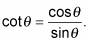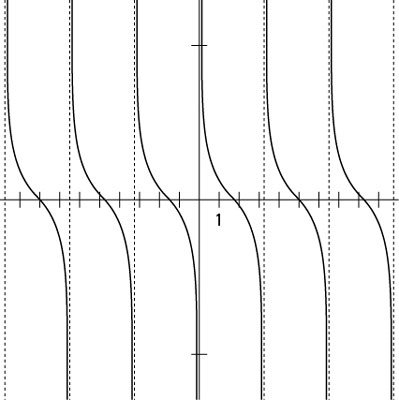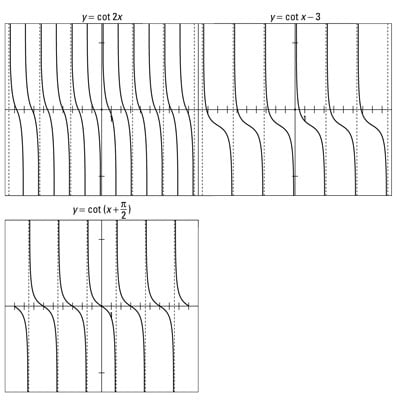##### Trigonometry Workbook For DummiesThe graphs of the tangent function lay the groundwork for the graphs of the cotangent function. After all, the tangent and cotangent are cofunctions and reciprocals, and have all sorts of connections.

The graphs of these two functions are similar in so many ways: They both have asymptotes crossing the graph at regular intervals, the graphs go from negative infinity to positive infinity in value, and they’re both affected by multiplying and adding. The biggest difference in the graphs is in the direction in which the graphs are drawn. The values of the tangent function appear to rise as you read from left to right. The function goes upward, disappears off the graph, and then reappears down below to start all over again. The cotangent function does the opposite — it appears to fall when you read from left to right.

The asymptotes of the cotangent curve occur where the sine function equals 0, becauseEquations of the asymptotes are of the form y = nπ, where n is an integer. Some examples of the asymptotes are y = –3π, y = –2π, y = –π, y = 0, y = π, y = 2π, and y =3π. The following figure shows the cotangent function graphed between –3π and 3π.The graph of the cotangent function.

Like the other functions, cotangent repeats the same values over and over. You can apply the same types of variations to cotangent that you can to tangent. The below figure depicts three examples of variations: multiplying the angle variable, subtracting from the function, and adding to the angle variable.Variations on the graph of the cotangent function.